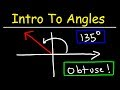# Free online courseTrigonometry by The Organic Chemistry Tutor

4.56

(16)

## 9h25m of online video course

Quality content and selected for your learning.

## Exercises to train your knowledge

Many of the courses available have exercises to help with learning.

This course is free today and always will be, from start to finish you will pay nothing.Teacher

##### The Organic Chemistry Tutor
###### 1 Courses / 143 students

This channel focuses on providing tutorial videos on organic chemistry, general chemistry, physics, algebra, trigonometry, precalculus, and calculus.

## Course content preview

There are no comments yet for this course.Watch the 1st video of the course

Do not worry, it is free!

## Course content0h14m

### Angles

This trigonometry video tutorial provides a basic introduction into angle measure. It explains the process of drawing angles in standard position in degrees. It discusses the difference between the initial side of an angle and its terminal side. In addition, it mentions the difference between an acute angle, right angle, obtuse angle, and a straight angle. This video contains plenty of examples and practice problems including drawing positive and negative angles in standard position.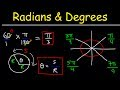0h18m

This video also explains how to draw angles in standard position using radians instead of degrees. It explains the location of common angles such as pi/4, 3pi/4, 5pi/4, and 7pi/4 and more. This video contains a lot of examples and practice problems.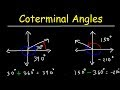0h06m

### Coterminal Angles In Radians & Degrees - Basic Introduction, Trigonometry

This trigonometry video tutorial provides a basic introduction into coterminal angles. It explains how to find coterminal angles of other angles in radians and degrees.

New Trigonometry Playlist:

https://www.patreon.com/MathScienceTutor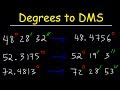0h11m

### Decimal Degrees to DMS Formula - Converting Degrees Minutes and Seconds to Decimal - Trigonometry

This trigonometry video tutorial explains how to convert decimal degrees to DMS. It also explains the process of converting DMS degrees minutes and seconds to decimal degrees. This video contains plenty of examples and practice problems for you to master the concept.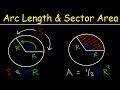0h15m

### Arc Length of a Circle Formula - Sector Area, Examples, Radians, In Terms of Pi, Trigonometry

This geometry and trigonometry video tutorial explains how to calculate the arc length of a circle using a formula given the angle in radians the and the length of the radius. It also explains how to calculate the sector area in terms of pi or in radians. In addition, it also explains how to determine the area of a sector of a circle using an equation where the angle is given in degrees instead of radians. This video contains plenty of examples and practice problems.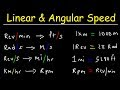0h18m

### Linear Speed and Angular Velocity

This trigonometry video tutorial provides a basic introduction into linear speed and angular velocity. It discusses how to convert linear speed to angular speed. It explains how to convert rpm values or revolutions per minute into feet per second. It also shows you how to convert rad/s to m/s, rev/s to mph, km/hr to rpm, and miles per hour to radians per second. This video contains plenty of examples and practice problems.

New Trigonometry Playlist:

https://www.patreon.com/MathScienceTutor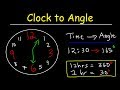0h12m

### Clock Aptitude Reasoning Tricks & Problems - Finding Angle Between The Hands of a Clock Given Time

This geometry & Trigonometry video tutorial explains how to solve clock aptitude problems with shortcuts and tricks provided. It explains how to find the angle between the hands of a clock given time. It's important to understand that 12 hours represent 360 degrees and 1 hour represents 30 degrees. If the minute hand is at 12, the hour hand will be directly on the number it's pointing at. If the minute hand is on the 6, the hour hand will be in between two numbers. This video contains plenty of examples and practice problems.

Geometry Playlist:

https://www.patreon.com/MathScienceTutor

Trigonometry Course: https://www.udemy.com/trigonometry-the-unit-circle-angles-right-triangles/learn/v4/overview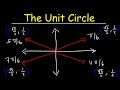0h12m

### The Unit Circle, Basic Introduction, Trigonometry

This trigonometry video tutorial provides a basic introduction into the unit circle. It explains how to evaluate trigonometric functions such as sine and cosine using the circle regardless if the angle is in radians or degrees. You can find the exact value of a trigonometric function if you have access to the unit circle.

New Trigonometry Playlist:

https://www.patreon.com/MathScienceTutor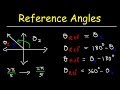0h12m

### How To Find The Reference Angle In Radians and Degrees - Trigonometry

This trigonometry video tutorial provides a basic introduction into reference angles. It explains how to find the reference angle in radians and degrees.

New Trigonometry Playlist:

https://www.patreon.com/MathScienceTutor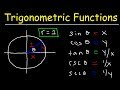0h02m

### The Six Trigonometric Functions, Basic Introduction, Trigonometry

This trigonometry video tutorial provides a basic introduction into the six trigonometric functions such as sine, cosine, tangent, cotangent, secant, and cosecant. It provides the equations and formulas associated with these trigonometric functions as they relate to the unit circle.

New Trigonometry Playlist:

https://www.patreon.com/MathScienceTutor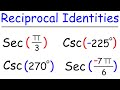0h16m

### Reciprocal Identities, Example Problems, Trigonometry, Evaluating Secant and Cosecant Functions

This trigonometry video tutorial explains how to use the reciprocal identities to evaluate trigonometric functions such as secant and cosecant. This video contains plenty of practice example problems.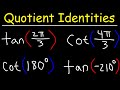0h12m

### Quotient Identities Trig Example, Evaluating Tangent and Cotangent Trigonometric Functions

This trigonometry video tutorial explains how to evaluate tangent and cotangent trigonometric functions using the quotient identities of tan and cot. This trig video contains plenty of examples and practice problems.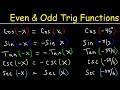0h10m

### Even and Odd Trigonometric Functions & Identities - Evaluating Sine, Cosine, & Tangent

This trigonometry video tutorial explains how to use even and odd trigonometric identities to evaluate sine, cosine, and tangent trig functions. This video contains plenty of examples and practice problems.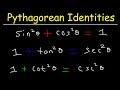0h07m

### Pythagorean Identities - Examples & Practice Problems, Trigonometry

This trigonometry video tutorial provides a basic introduction into the pythagorean identities of trigonometric functions. it provides plenty of examples and practice problems of evaluating cosine given the value of sine.

New Trigonometry Playlist:

https://www.patreon.com/MathScienceTutor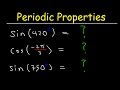0h04m

### How To Evaluate Trigonometric Functions Using Periodic Properties - Trigonometry

This trigonometry video tutorial explains how to evaluate trigonometric functions using periodic properties of sine and cosine in radians and degrees.

New Trigonometry Playlist:

https://www.patreon.com/MathScienceTutor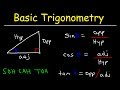0h21m

### Trigonometry For Beginners!

This math video tutorial provides a basic introduction into trigonometry. It covers trigonometric ratios such as sine, cosine, and tangent. It explains how to evaluate it using right triangle trigonometry and SOHCAHTOA. In addition, it explains how to solve the missing sides of triangles and how to find the missing angles using inverse trig functions. This video contains useful trigonometry lessons for beginners. it contains plenty of examples and practice problems.

Trigonometry Online Course:
https://www.udemy.com/trigonometry-the-unit-circle-angles-right-triangles/

Algebra Online Course:
https://www.udemy.com/algebracourse7245/learn/v4/overview

https://www.patreon.com/MathScienceTutor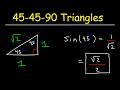0h02m

### 45-45-90 Triangles, Special Right Triangle Trigonometry

This trigonometry video tutorial provides a basic introduction into 45-45-90 triangles. It explains how to evaluate trigonometric functions such as sine and cosine using special reference triangles.

New Trigonometry Playlist:

https://www.patreon.com/MathScienceTutor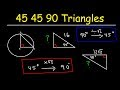0h10m

### 45-45-90 Triangles For SAT & ACT Math - Trigonometry & Geometry

This video tutorial provides a basic introduction into 45-45-90 right triangles and explains how to use this special reference triangle to find the value of the missing sides of another triangle. This information is useful for students taking trigonometry or geometry in high school and college and for those studying the sat math and act math sections. This video contains plenty of examples and practice problems.

New Trigonometry Playlist:

https://www.patreon.com/MathScienceTutor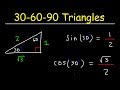0h03m

### 30-60-90 Triangles - Special Right Triangle Trigonometry

This trigonometry video tutorial provides a basic introduction into 30-60-90 triangles. It explains how to evaluate trigonometric functions such as sine and cosine using right triangle trigonometry.

New Trigonometry Playlist:

https://www.patreon.com/MathScienceTutor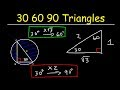0h11m

### 30-60-90 Special Right Triangles For ACT & SAT Math - Geometry & Trigonometry

This video tutorial provides a basic introduction into 30-60-90 triangles. It explains how to find the value of the missing side of other triangles using the 30-60-90 reference triangle. This information is useful for high school and college students studying geometry and trigonometry and for students studying the SAT math and ACT math sections. This tutorial contains plenty of examples and practice problems.

New Trigonometry Playlist: